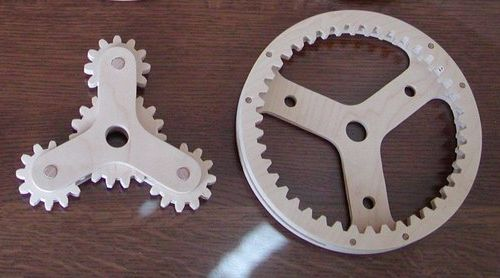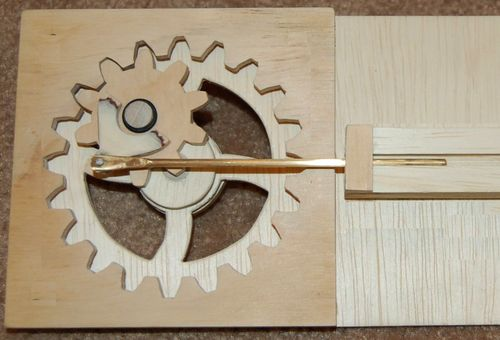# Planetary gear ratio calculations

A question that I often get is how to work out planetary gears using the gear template generatorWorking out the tooth counts for planetary gears is actually not that complicated, so I initially neglected to mention how to do it. But having received the question a number of times, I'll elaborate.

For convenience, let's denote R, S, and P as the number of teeth on the gears.

 R Number of teeth in ring gear S Number of teeth in sun (middle) gear P Number of teeth in planet gears
The first constraint for a planetary gear to work out is that all teeth have the same pitch, or tooth spacing. This ensures that the teeth mesh.

The second constraint is:
R = 2 × P + S

That is to say, the number of teeth in the ring gear is equal to the number of teeth in the middle sun gear plus twice the number of teeth in the planet gears.

In the gear at left, this would be 30 = 2 × 9 + 12This can be made more clear by imagining "gears" that just roll (no teeth), and imagine an even number of planet gears. From the illustration at left, you can see that the diameters of the sun gear, plus two planet gears be must equal to the ring gear size.

Now imagine we take out one of the green planet wheels, and rearrange the remaining ones to be evenly spaced. Still the same size gear.

Now imagine the wheels have teeth. The teeth would stick out beyond the line of the wheel as much as they indent, so that the pitch line of the gears would be the line around the gears. The geometry still works the same. If you go into the gear generator and select "show pitch diameter", you can see how the pitch diameter is just a circle that the teeth are centered over.

The pitch diameter of a gear is just the number of teeth divided by diametrical pitch (larger values of "diametrical pitch" mean smaller teeth). The gear generator program tends to refer to tooth spacing. Pitch diameter can also be calculated as tooth spacing * number of teeth / (2*π), where 2*π = 6.283Here's another planetary gear set. The middle arrangement is removed...... and here it is inserted.

In this case, the planet gears have 12 teeth, the sun gear has 18 and the ring gear has 42 teeth.

So, applying

R = 2×P + S

We get

42 = 2 × 12 + 18

These pictures are part of a fasincatingly complicated planetary gear drive by Ronald Walters.

### Working out planetary gear turns ratios

Working out the gear ratio of a planetary gear train can be tricky. Let's denote the following:
 Tr Turns of the ring gear Ts Turns of the sun gear Ty Turns of the planetary gear carrier (the Y shaped thing in the previous photo) R Ring gear teeth S Sun gear teeth P Planet gear teeth
The turns ratio is as follows:

( R + S ) ×Ty = R × Tr + Ts × S

#### Example:

Now, usually in a planetary gear, one of the gears is held fixed. For example, if we hold the ring gear in a fixed position, Tr will always be zero. So we can remove those terms from the above formula, and we get:

( R + S ) × Ty = Ts × S

Now, if we drive the sun gear, we can rearrange the formula to solve for turns of the Y carrier:

 Ty = Ts× S R+S
So the gear ratio is

S / (R+S)

### Constraints on number of teeth and planets

If you want the planetary gears to be evenly spaced, and all be engaging the next tooth at the same time, then both your sun and your ring gear needs to be evenly divisible by the number of planets.

If you want them to be evenly spaced, but don't need them to all be in the same phase with respect to their teeth, then then the sum of the ring gear's teeth and the sun gear's teeth must be evenly divisible by the number of planets. That is:

( R + S ) is evenly divides by the number of planets.

If however you are willing to space the planets unevenly, this constraint does not apply. The angle between the planet gears about the sun gear is however still constrained by:

 Anglep2p = 360 ×N Where N is a whole number R+S
That is to say, the angle between planet gears is a multiple of 360/(R+S).Finally, here's another cool arrangement of gears, though not really a "planetary" gear set.

If you place a gear inside another gear, with the internal gear having a tooth count of half the ring gear's tooth count, any point on the pitch diameter of the inside gear will move back and forth in a straight line.

The brass rod in this photo will move strictly left to right in the slot, while the gear it is attached to rolls around inside the ring gear. That gear is actually attached to a crank which keeps it rolling around the edge, although only the center part of that crank is visible, so it doesn't really look like a crank in the photo.

Photo credits:
I used some photos that were sent to me by readers. The first and last photos were taken by Brian Kerr. The second and third photos were sent to me by Ronald Walters